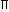# Aptitude - Volume and Surface Area

Exercise : Volume and Surface Area - General Questions
11.
The curved surface area of a cylindrical pillar is 264 m2 and its volume is 924 m3. Find the ratio of its diameter to its height.
3 : 7
7 : 3
6 : 7
7 : 6
Explanation:r2h = 924r =924 x 2= 7 m. 2rh 264 264

 And, 2rh = 264h =264 x 7 x 1 x 1= 6m. 22 2 7Required ratio = 2r = 14 = 7 : 3. h 6

12.
A cistern of capacity 8000 litres measures externally 3.3 m by 2.6 m by 1.1 m and its walls are 5 cm thick. The thickness of the bottom is:
90 cm
1 dm
1 m
1.1 cm
Explanation:

Let the thickness of the bottom be x cm.

Then, [(330 - 10) x (260 - 10) x (110 - x)] = 8000 x 1000320 x 250 x (110 - x) = 8000 x 1000(110 - x) = 8000 x 1000 = 100 320 x 250x = 10 cm = 1 dm.

13.
What is the total surface area of a right circular cone of height 14 cm and base radius 7 cm?
344.35 cm2
462 cm2
498.35 cm2
None of these
Explanation:

h = 14 cm, r = 7 cm.

So, l = (7)2 + (14)2 = 245 = 75 cm.Total surface area =rl +r2
 =22 x 7 x 75 + 22 x 7 x 7cm2 7 7
= [154(5 + 1)] cm2
= (154 x 3.236) cm2
= 498.35 cm2.

14.
A large cube is formed from the material obtained by melting three smaller cubes of 3, 4 and 5 cm side. What is the ratio of the total surface areas of the smaller cubes and the large cube?
2 : 1
3 : 2
25 : 18
27 : 20
Explanation:

Volume of the large cube = (33 + 43 + 53) = 216 cm3.

Let the edge of the large cube be a.

So, a3 = 216a = 6 cm.Required ratio =6 x (32 + 42 + 52)= 50 = 25 : 18. 6 x 62 36

15.
How many bricks, each measuring 25 cm x 11.25 cm x 6 cm, will be needed to build a wall of 8 m x 6 m x 22.5 cm?
5600
6000
6400
7200
 Number of bricks = Volume of the wall =800 x 600 x 22.5= 6400. Volume of 1 brick 25 x 11.25 x 6